Important Surface Area Questions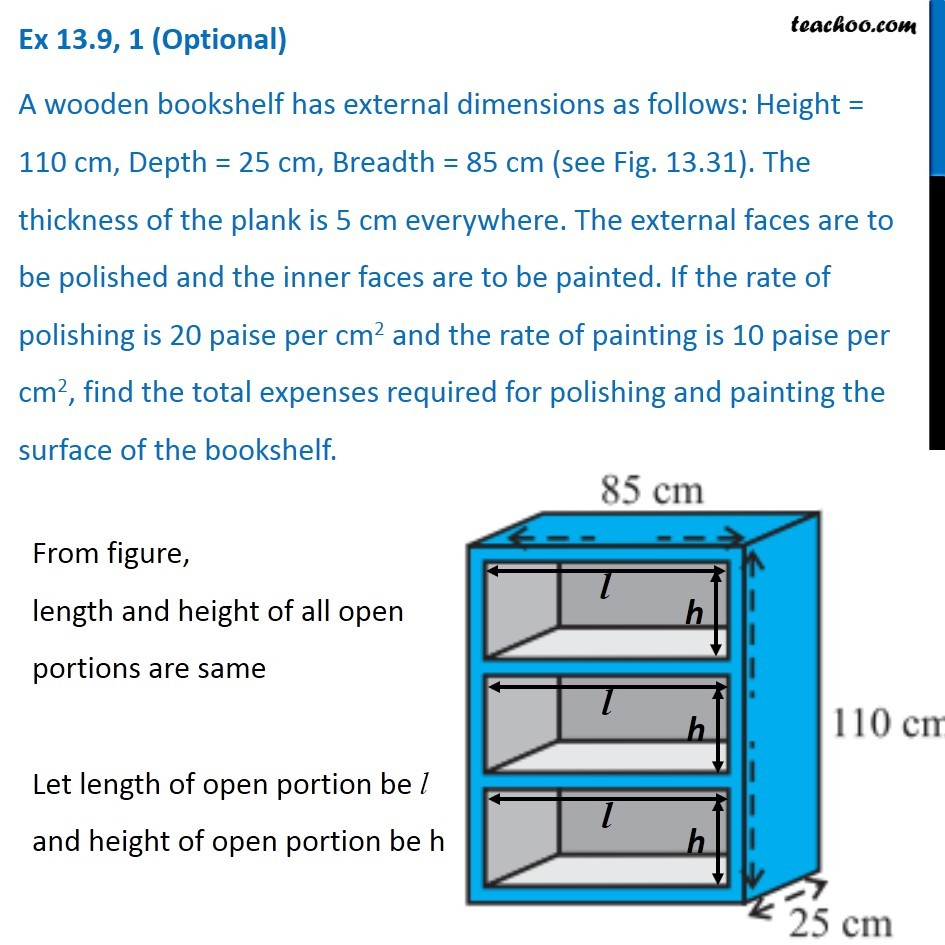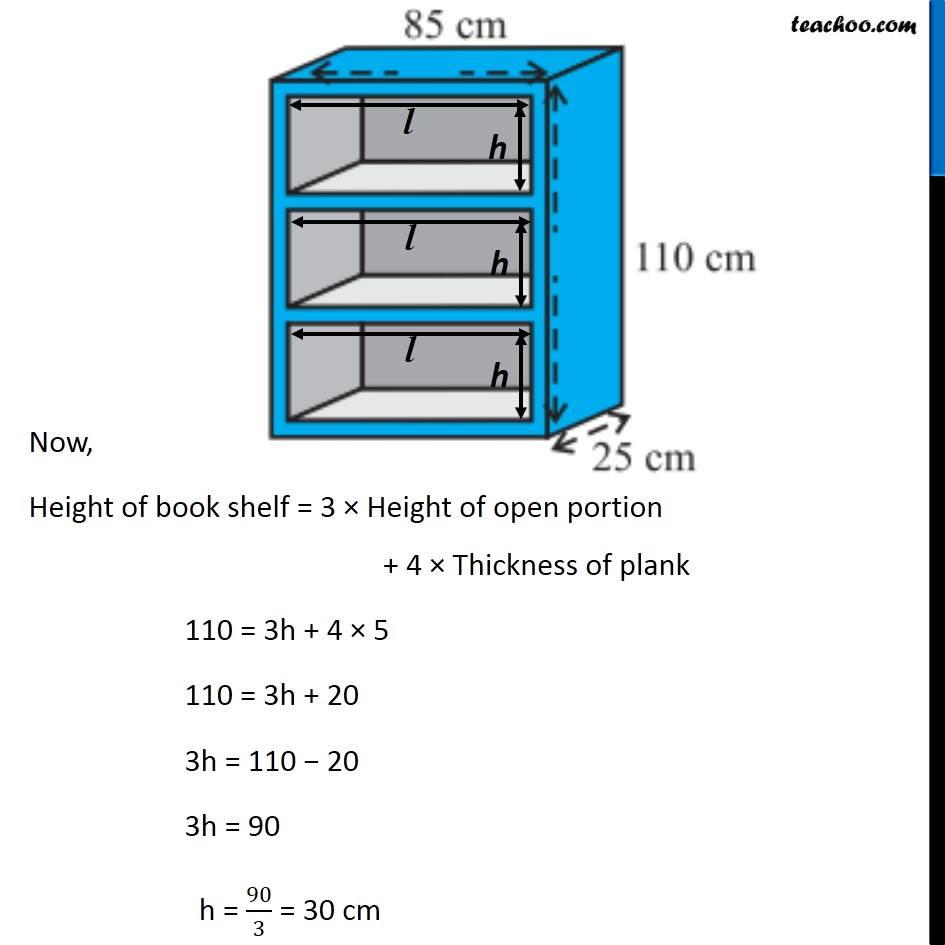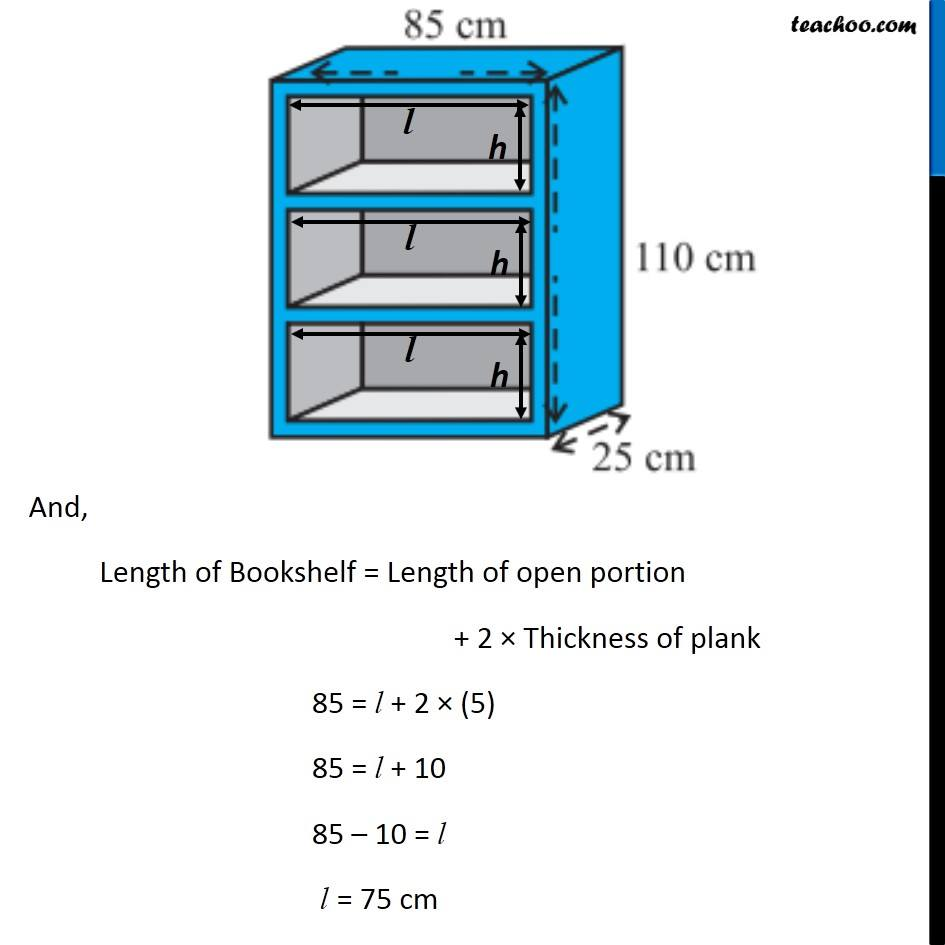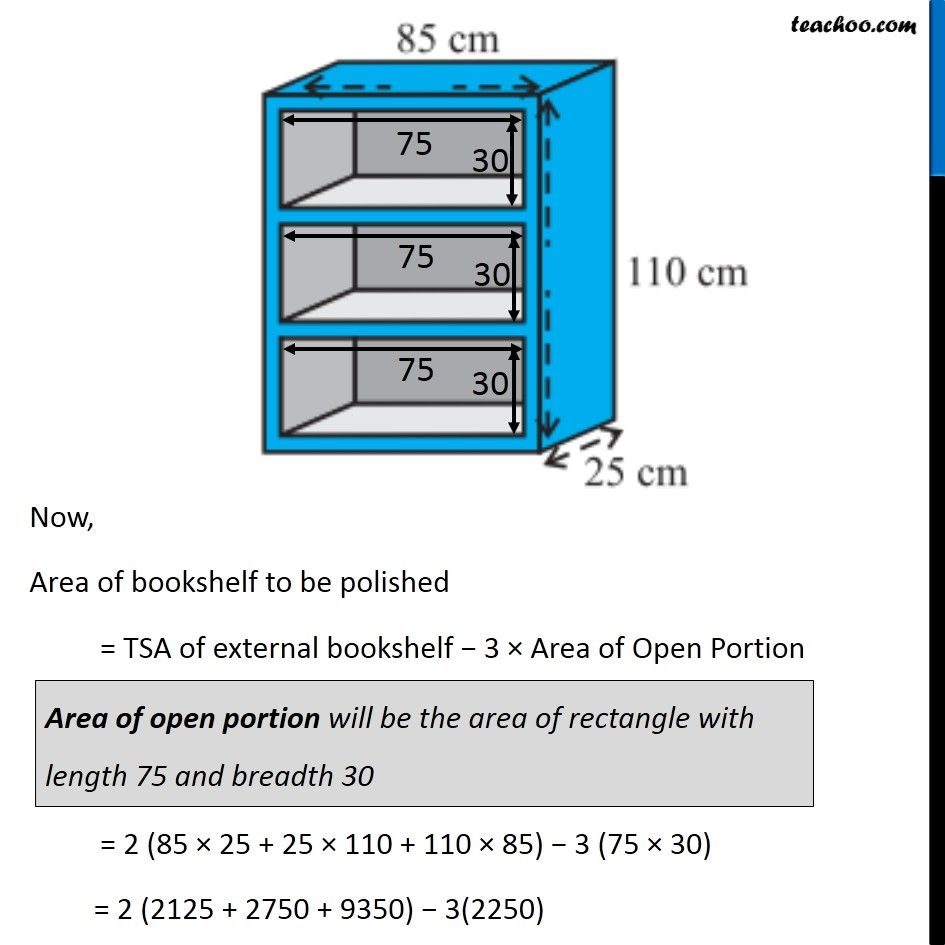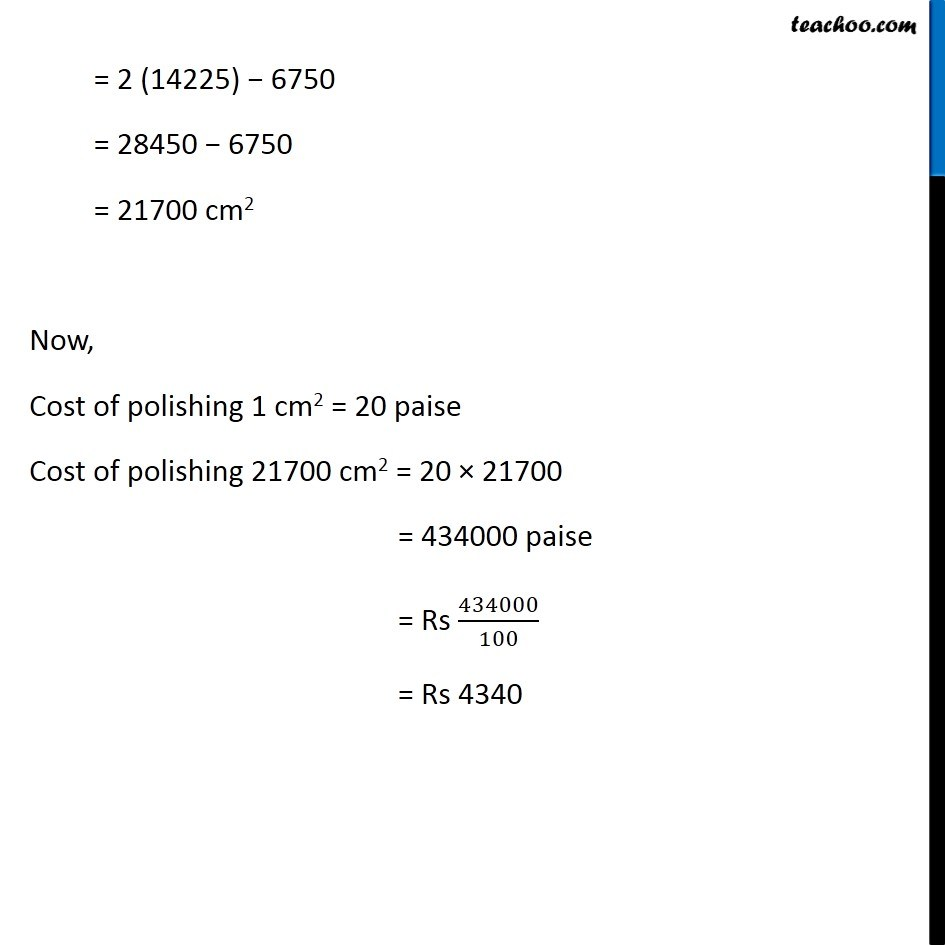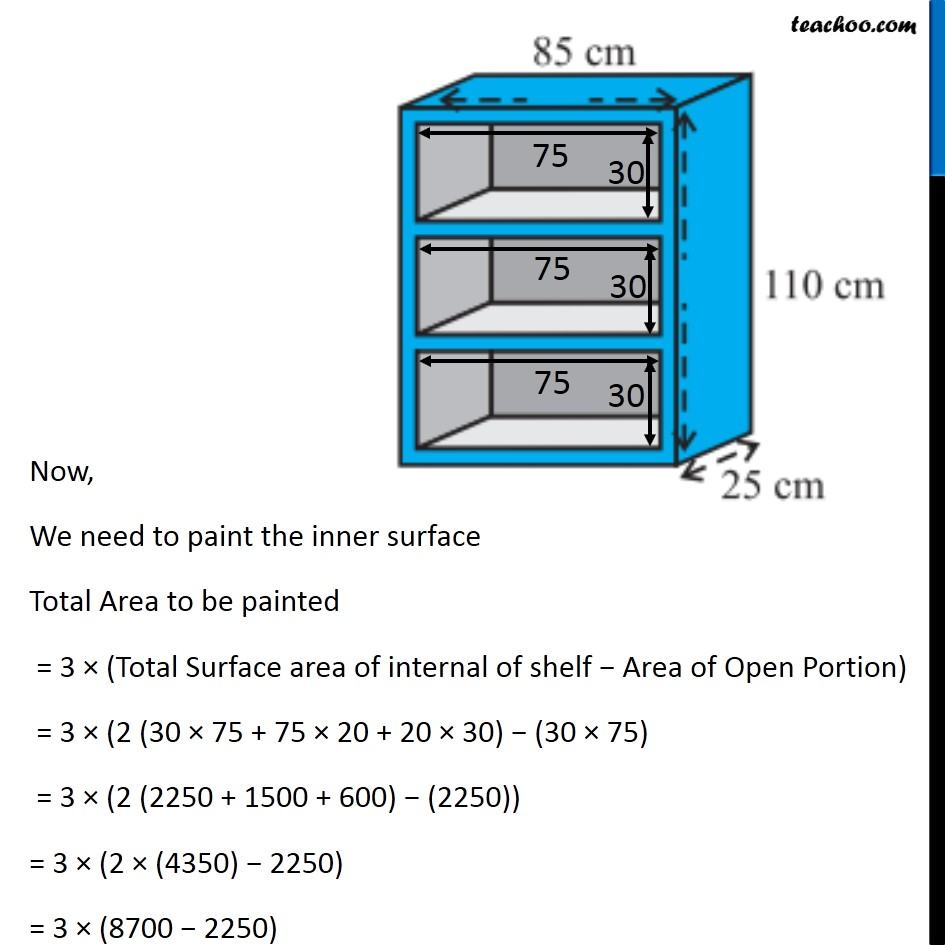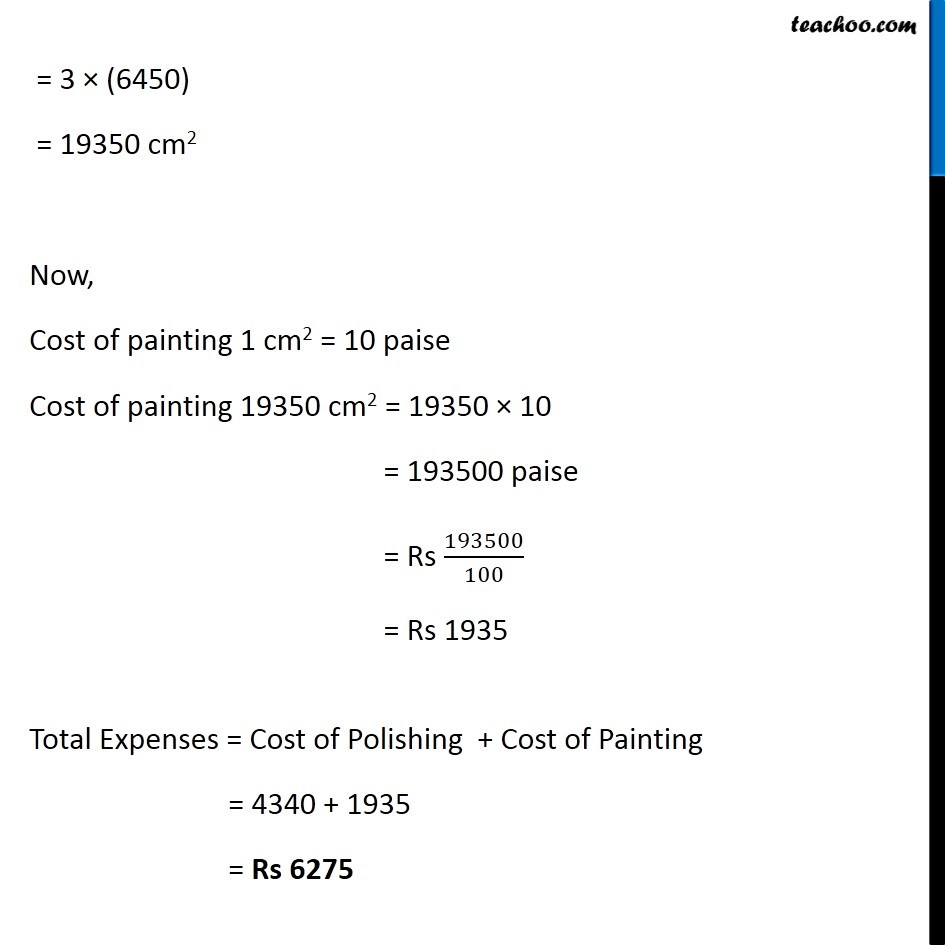Learn in your speed, with individual attention - Teachoo Maths 1-on-1 Class

### Transcript

Question 1 A wooden bookshelf has external dimensions as follows: Height = 110 cm, Depth = 25 cm, Breadth = 85 cm (see Fig. 13.31). The thickness of the plank is 5 cm everywhere. The external faces are to be polished and the inner faces are to be painted. If the rate of polishing is 20 paise per cm2 and the rate of painting is 10 paise per cm2, find the total expenses required for polishing and painting the surface of the bookshelf. From figure, length and height of all open portions are same Let length of open portion be l and height of open portion be h Now, Height of book shelf = 3 × Height of open portion + 4 × Thickness of plank 110 = 3h + 4 × 5 110 = 3h + 20 3h = 110 − 20 3h = 90 h = 90/3 = 30 cm And, Length of Bookshelf = Length of open portion + 2 × Thickness of plank 85 = l + 2 × (5) 85 = l + 10 85 – 10 = l l = 75 cm Now, Area of bookshelf to be polished = TSA of external bookshelf − 3 × Area of Open Portion Area of open portion will be the area of rectangle with length 75 and breadth 30 Area of open portion will be the area of rectangle with length 75 and breadth 30 = 2 (14225) − 6750 = 28450 − 6750 = 21700 cm2 Now, Cost of polishing 1 cm2 = 20 paise Cost of polishing 21700 cm2 = 20 × 21700 = 434000 paise = Rs 434000/100 = Rs 4340 Now, We need to paint the inner surface Total Area to be painted = 3 × (Total Surface area of internal of shelf − Area of Open Portion) = 3 × (2 (30 × 75 + 75 × 20 + 20 × 30) − (30 × 75) = 3 × (2 (2250 + 1500 + 600) − (2250)) = 3 × (2 × (4350) − 2250) = 3 × (8700 − 2250) = 3 × (6450) = 19350 cm2 Now, Cost of painting 1 cm2 = 10 paise Cost of painting 19350 cm2 = 19350 × 10 = 193500 paise = Rs 193500/100 = Rs 1935 Total Expenses = Cost of Polishing + Cost of Painting = 4340 + 1935 = Rs 6275